# MATLAB04:基础绘图

## 一.图线的绘制与装饰

### 1.使用plot()函数绘制图线

plot(x,y,LineSpec)

• x: 图线上点的x坐标
• y: 图线上点的y坐标
• LineSpec: 图线的线条设定,三个指定线型,标记符号颜色设定符组成一个字符串,设定符不区分先后.具体细节请参考官方文档.

| 线型符号 | 线型设定符 | 标记 | 标记设定符 | 颜色 | 颜色设定符 |
| :------: | :----------: | :--: | :--------: | :--: | :--------: |
| - | 实线（默认） | o | 圆圈 | y | 黄色 |
| -- | 虚线 | + | 加号 | m | 品红色 |
| -- | 点线 | * | 星号 | c | 青蓝色 |
| -. | 点划线 | . | 点 | r | 红色 |
| | | x | 叉号 | g | 绿色 |
| | | s | 方形 | b | 蓝色 |
| | | d | 菱形 | w | 白色 |
| | | ^ | 上三角 | k | 黑色 |
| | | v | 下三角 | | |
| | | > | 右三角 | | |
| | | < | 左三角 | | |
| | | p | 五角形 | | |
| | | h | 六角形 | | |

x = 0:pi/20:2*pi;
y = cos(x);
plot(x, y, 'r.-')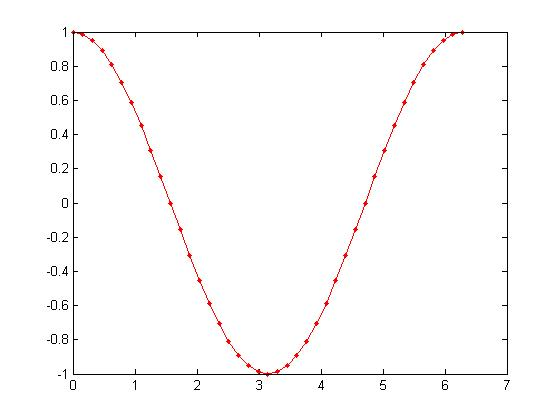### 2.装饰图线

• 使用legend()函数为图片增加图例

x=0:0.5:4*pi;
y=sin(x); h=cos(x); w=1./(1+exp(-x)); g=(1/(2*pi*2)^0.5).*exp((-1.*(x-2*pi).^2)./(2*2^2));
plot(x,y,'bd-' ,x,h,'gp:',x,w,'ro-' ,x,g,'c^-');        % 绘制多条图线
legend('sin(x)','cos(x)','Sigmoid','Gauss function');    % 添加图例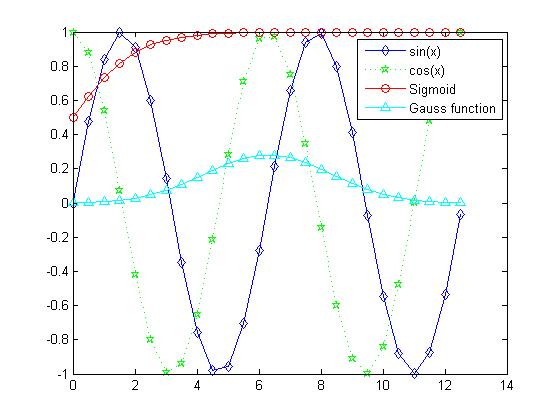• 使用title()*label()为图片增加标题和标签

x = 0:0.1:2*pi; y1 = sin(x); y2 = exp(-x);
plot(x, y1, '--*', x, y2, ':o');
xlabel('t = 0 to 2\pi');
ylabel('values of sin(t) and e^{-x}')
title('Function Plots of sin(t) and e^{-x}');
legend('sin(t)','e^{-x}');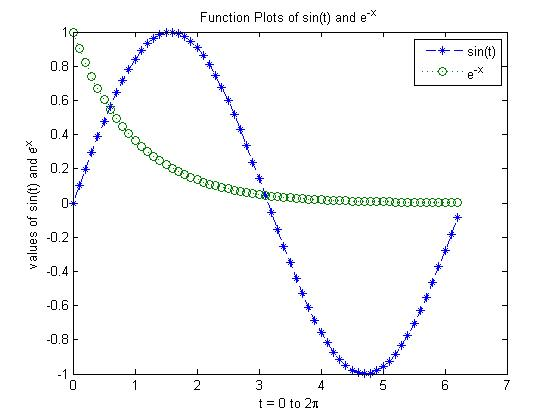• 使用text()annotation()为图片增加注解

x = linspace(0,3); y = x.^2.*sin(x); plot(x,y);
line([2,2],[0,2^2*sin(2)]);
str = '$$\int_{0}^{2} x^2\sin(x) dx$$';
text(0.25,2.5,str,'Interpreter','latex');
annotation('arrow','X',[0.32,0.5],'Y',[0.6,0.4]);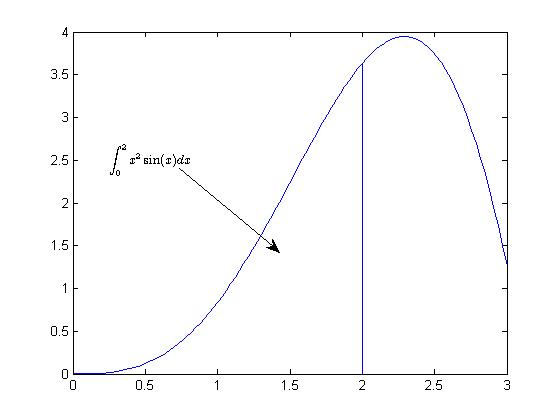### 3.控制坐标轴,边框与网格

grid on/off设置网格可见性
box on/off设置边框可见性
axis on/off设置坐标轴可见性
axis normal还原默认行为,将图框纵横比模式和数据纵横比模式的属性设置为自动
axis square使用相同长度的坐标轴线,相应调整数据单位之间的增量
axis equal使用相同长度的坐标轴线,相应调整数据单位之间的增量
axis tight将坐标轴范围设置为等同于数据范围,使轴框紧密围绕数据

t = 0:0.1:2*pi; x = 3*cos(t); y = sin(t);
subplot(2, 2, 1); plot(x, y); axis normal
subplot(2, 2, 2); plot(x, y); axis square
subplot(2, 2, 3); plot(x, y); axis equal
subplot(2, 2, 4); plot(x, y); axis equal tight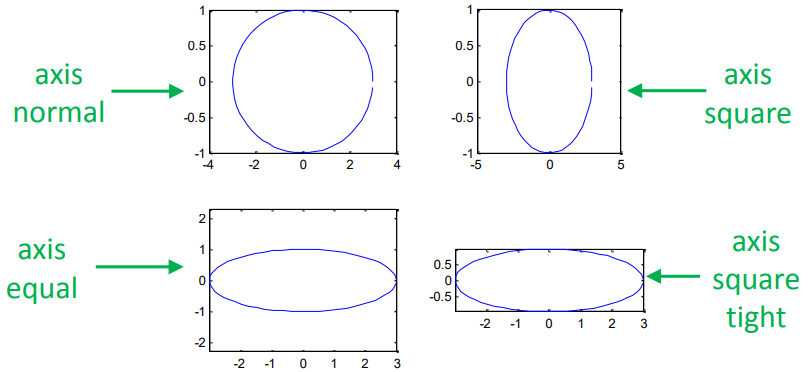## 二.绘制多条图线

### 1.在一个图像上绘制多条图线

plot(cos(0:pi/20:2*pi));
plot(sin(0:pi/20:2*pi));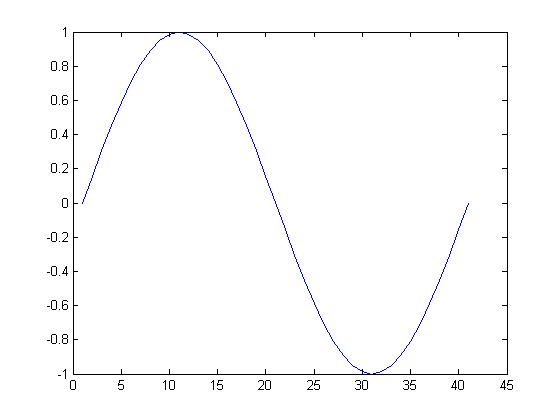hold on        % 提起画笔,开始绘制一组图片
plot(cos(0:pi/20:2*pi));
plot(sin(0:pi/20:2*pi));
hold off    % 放下画笔,该组图片绘制完毕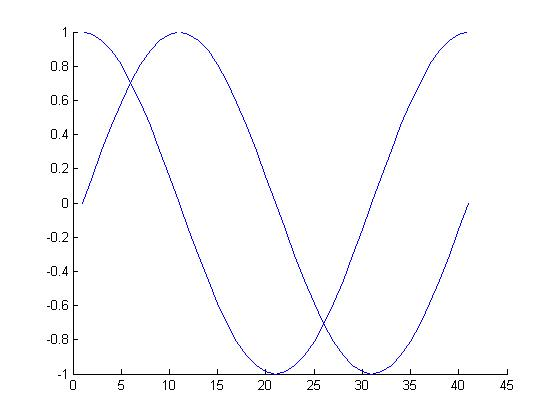### 2.在一个窗口内绘制多个图像

subplot(m,n,p)

subplot(2,2,1);
x = linspace(-3.8,3.8);
y_cos = cos(x);
plot(x,y_cos);
title('Subplot 1: Cosine')

subplot(2,2,2);
y_poly = 1 - x.^2./2 + x.^4./24;
plot(x,y_poly,'g');
title('Subplot 2: Polynomial')

subplot(2,2,[3,4]);
plot(x,y_cos,'b',x,y_poly,'g');
title('Subplot 3 and 4: Both')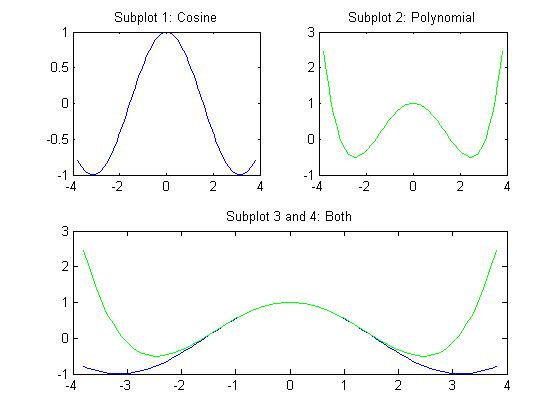## 三.图形对象的操作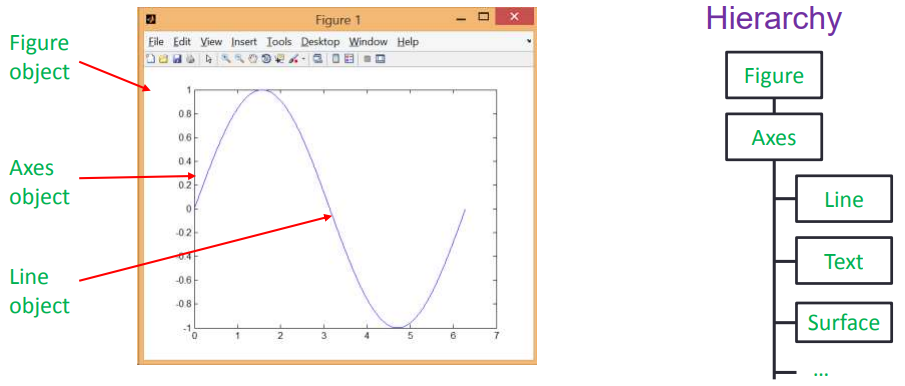### 1.获取图形句柄

FunctionPurpose
gca()获取当前坐标轴的句柄
gcf()获取当前图像的句柄
allchild(handle_list)获取该对象的所有子对象的句柄
ancestor(h,type)获取对象最近的type类型的祖先节点
delete(h)删除某对象
findall(handle_list)获取该对象的后代对象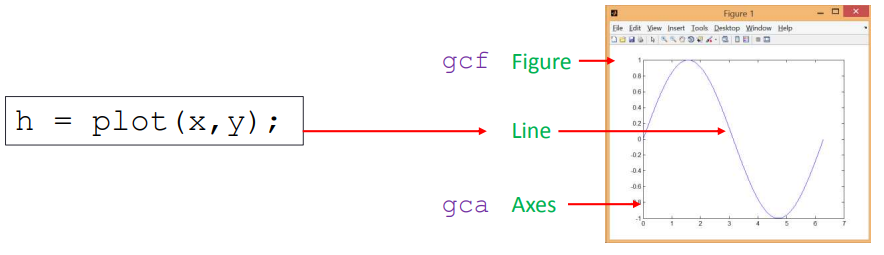### 2.通过图形句柄操作图形属性

• set(H,Name,Value)
• v = get(h,propertyName)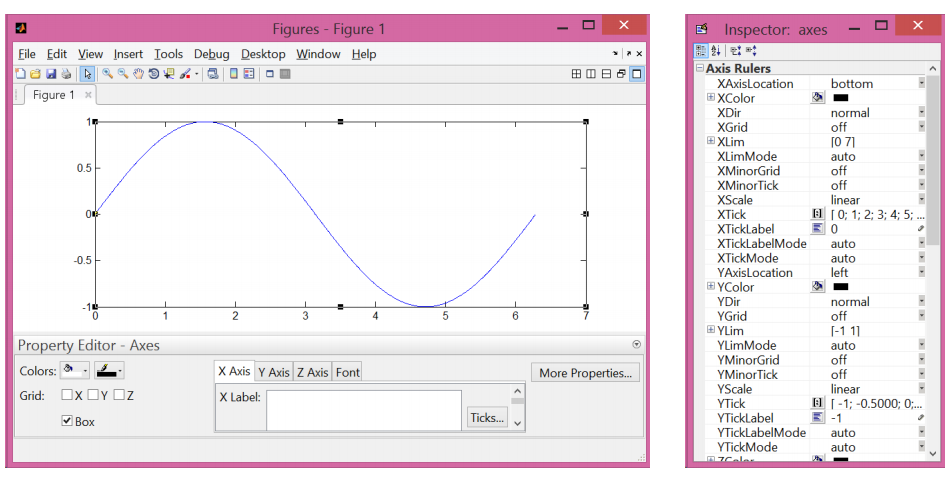1. 改变坐标轴属性:

% 第一张图
set(gca, 'FontSize', 25);

% 第二张图
set(gca, 'XTick', 0:pi/2:2*pi);
set(gca, 'XTickLabel', 0:90:360);

% 第三张图
set(gca, 'FontName', 'symbol');
set(gca, 'XTickLabel', {'0', 'p/2', 'p', '3p/2', '2p'});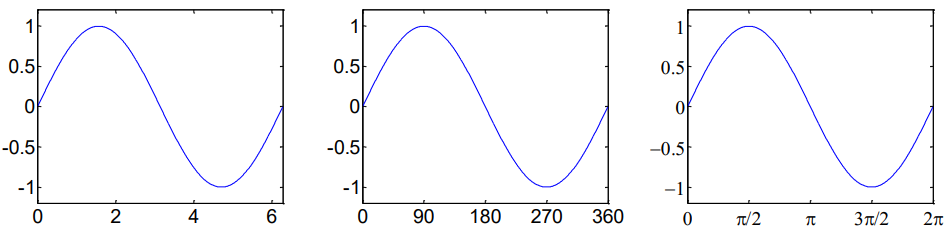1. 改变线型

h = plot(x,y);
set(h, 'LineStyle','-.', ...
'LineWidth', 7.0, ...
'Color', 'g');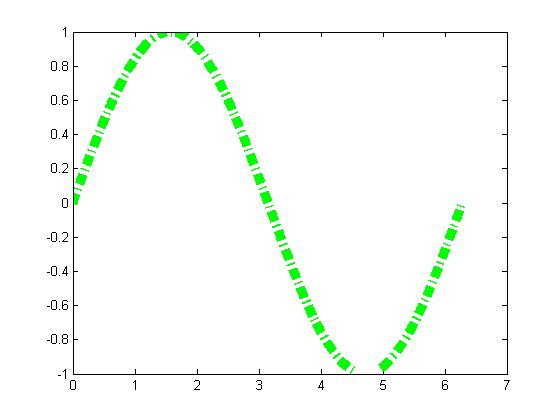## 三.将图形保存到文件

saveas(gcf, 'myfigure.png')